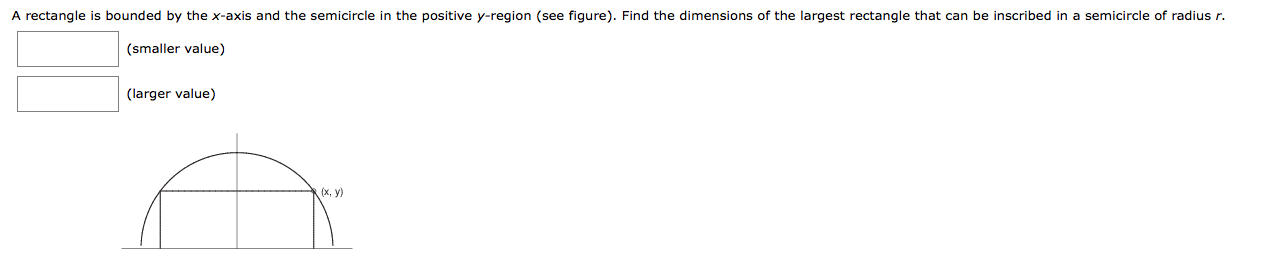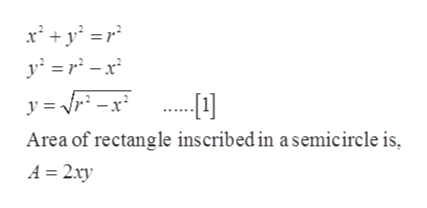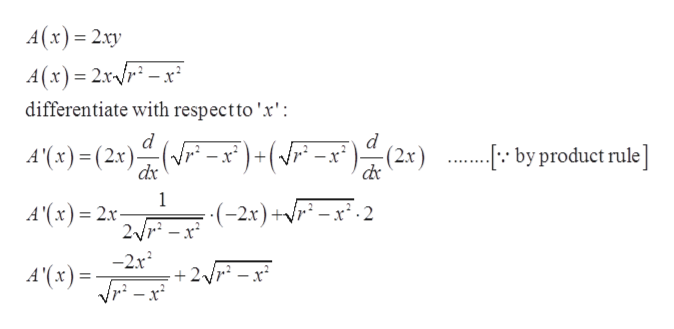# A rectangle is bounded by the x-axis and the semicircle in the positive y-region (see figure). Find the dimensions of the largest rectangle that can be inscribed in a semicircle of radius r.(smaller value)(larger value)(x, y

Question
6 views

A rectangle is bounded by the x-axis and the semicircle in the positive y-region (see figure). Find the dimensions of the largest rectangle that can be inscribed in a semicircle of radius r.help_outlineImage TranscriptioncloseA rectangle is bounded by the x-axis and the semicircle in the positive y-region (see figure). Find the dimensions of the largest rectangle that can be inscribed in a semicircle of radius r. (smaller value) (larger value) (x, y fullscreen
check_circle

Step 1

Given:

Assume that the center of the semicircle be (0,0).

Step 2

Concept used:

The equation of the semicircle is given:help_outlineImage Transcriptionclosey r* =x° .. Area of rectangle inscribed in a semicircle is, A 2xy fullscreen
Step 3

Substitute the value of ‘y’ in Area...help_outlineImage TranscriptioncloseA(x) 2xy A (x) 2x-r-x differentiate with respectto'x': d A(x)(2x) ( (2x) by produet rule] +(vr° -x : by product rule] dx 1 (-2x)+r-x2 A'(x)= 2x- 2/r-x -2x2 +2/r -x° A'(x) fullscreen

### Want to see the full answer?

See Solution

#### Want to see this answer and more?

Solutions are written by subject experts who are available 24/7. Questions are typically answered within 1 hour.*

See Solution
*Response times may vary by subject and question.
Tagged in

### Derivative